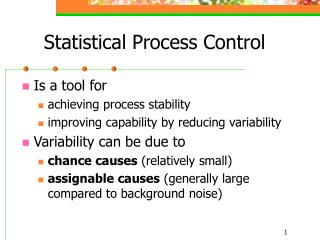DownloadDownload PresentationStatistical Process Control

# Statistical Process Control

Download Presentation## Statistical Process Control

- - - - - - - - - - - - - - - - - - - - - - - - - - - E N D - - - - - - - - - - - - - - - - - - - - - - - - - - -
##### Presentation Transcript

1. Statistical Process Control • Is a tool for • achieving process stability • improving capability by reducing variability • Variability can be due to • chance causes (relatively small) • assignable causes (generally large compared to background noise)

2. Control Charts • UCL = mW + ksW • CL = mW • LCL = mW – ksW UCL CL LCL • Types of Control Charts: • Variables Control Charts • Attributes Control Charts

3. Purpose of Control Charts • Improving productivity • Preventing defects • Preventing unnecessary process adjustments • Providing diagnostic information • Providing information about process capability

4. Design of a Control Chart • An control chart: • Standard deviation of the sample average. • Choosing the control limits. • Relationship to hypothesis testing. • Decisions: • Sample size to use. • Frequency of sampling.

5. Example of the control chart • In manufacturing automobile engine piston rings, the inside diameter of the rings is a critical quality characteristic. Given the process average diameter is 74mm, the process standard deviation of diameter is 0.01mm and the sample size is 5. • How the control limits are determined?

6. Analysis of Patterns • Types of patterns: • Run (8 or more) • Cyclic behavior • Western Electric Rules (Zone Rules): • One point outside 3s control limits. • 2 / 3 consecutive points beyond a 2s CL. • 4 / 5 consecutive points at 1s from CL. • 8 consecutive points on one side of the CL.

7. The Control Chart • When m and s are unknown… • R = Range of the sample • W = Relative range = R / s • E(W) = d2; Stdev(W) = d3 • What would be an estimator for s? • What are the UCL and LCL?

8. Definitions control chart R control chart

9. The R Control Chart • What is a reasonable estimator for sR? • What are the corresponding CL’s? • Example:

10. P Chart • where is the observed value of the average fraction defective

11. U Chart • where is the observed value of the average fraction defective

12. Control Chart Performance • ARL = Average Run Length • p -- probability that any point exceeds control limits